30 一月 2017, 09:14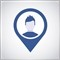0
12 726

### 1. 简介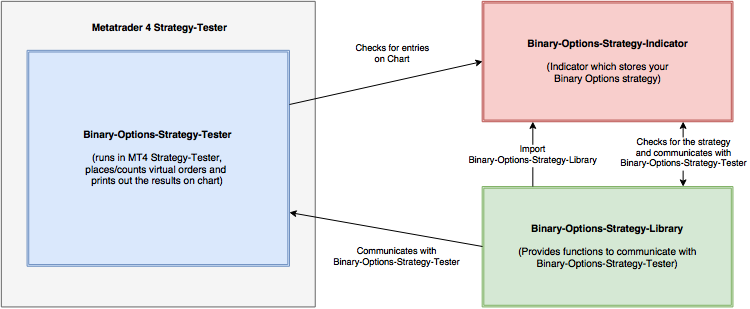### 2. 安装

KVO 指标是用作示例的，它展示了对外部指标和它们的 ex4 文件的访问，章节为 "3.2.6 使用外部指标 (ex4 文件)"。参见 https://www.mql5.com/en/code/8677 以获得指标的详细信息。

### 3.1定义二元期权策略

int ma_period
int ma_method
int applied_price

int period_fast        =  5;
int period_slow        = 10;
int method_both        =  0;
int applied_price_both =  0;

### 3.2创建二元期权策略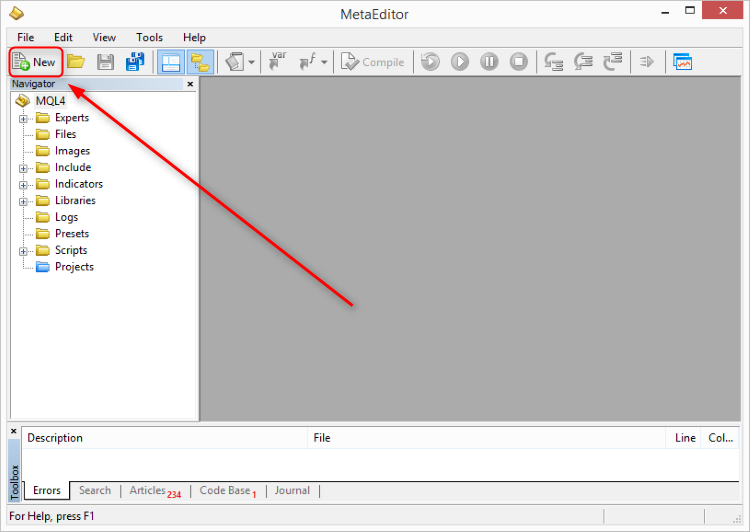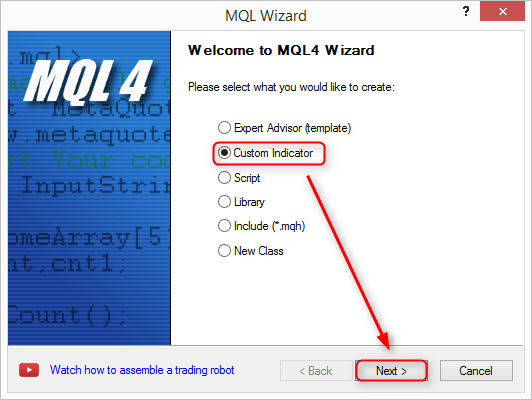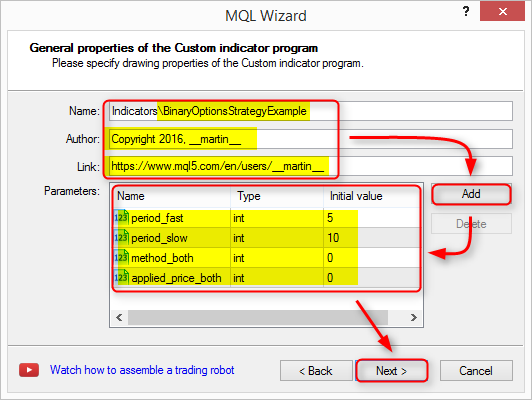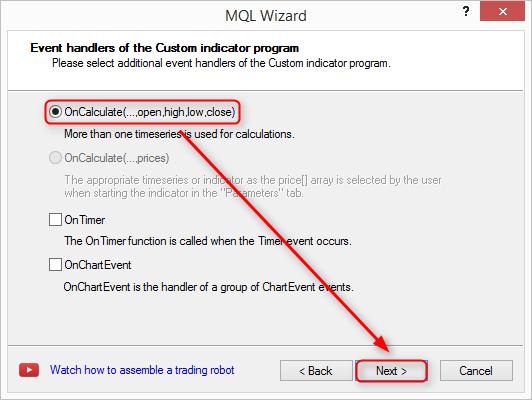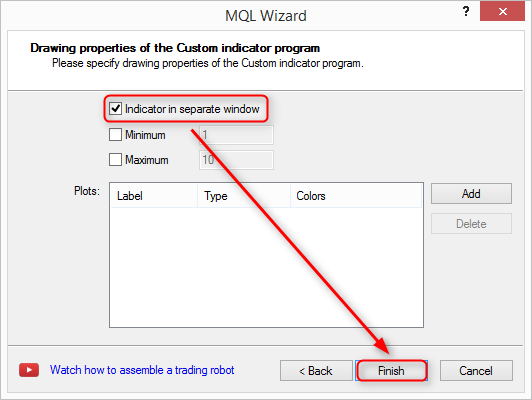//+------------------------------------------------------------------+
//|                                 BinaryOptionsStrategyExample.mq4 |
//|                         https://www.mql5.com/en/users/__martin__ |
//+------------------------------------------------------------------+
#property version   "1.00"
#property strict
#property indicator_separate_window
//--- 输入参数
input int      period_fast=5;
input int      period_slow=10;
input int      method_both=0;
input int      applied_price_both=0;
//+------------------------------------------------------------------+
//| 自定义指标初始化函数                        |
//+------------------------------------------------------------------+
int OnInit()
{
//--- 指标缓冲区映射

//---
return(INIT_SUCCEEDED);
}
//+------------------------------------------------------------------+
//| 自定义指标迭代函数                        |
//+------------------------------------------------------------------+
int OnCalculate(const int rates_total,
const int prev_calculated,
const datetime &time[],
const double &open[],
const double &high[],
const double &low[],
const double &close[],
const long &tick_volume[],
const long &volume[],
{
//---

//--- 返回 prev_calculated 的数值用于下一次调用
return(rates_total);
}
//+------------------------------------------------------------------+

### 3.2.1输入参数

...

//--- 输入参数
input int                period_fast        =  5;           //快速移动平均周期数
input int                period_slow        = 10;           //慢速移动平均周期数
input ENUM_MA_METHOD     method_both        = MODE_SMA;     //移动平均方法
input ENUM_APPLIED_PRICE applied_price_both = PRICE_CLOSE;  //移动平均应用的价格

...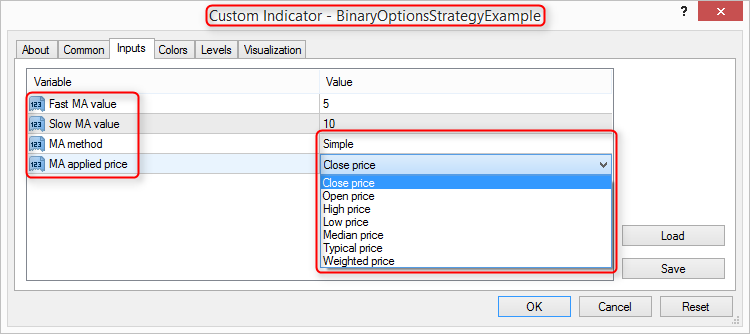### 3.2.2包含 Binary-Options-Strategy-Library

//+------------------------------------------------------------------+
//|                                 BinaryOptionsStrategyExample.mq4 |
//|                         https://www.mql5.com/en/users/__martin__ |
//+------------------------------------------------------------------+
#property version   "1.00"
#property strict
#property indicator_separate_window

#include <BinaryOptionsStrategyLibrary.mqh>

//--- 输入参数

...

Binary-Options-Strategy-Library 将会使用两个新的参数来加强输入参数:

• 每个烛形只能设置一个卖出或者一个买入交易；
• 策略中只有新的烛形开始时才进行检查。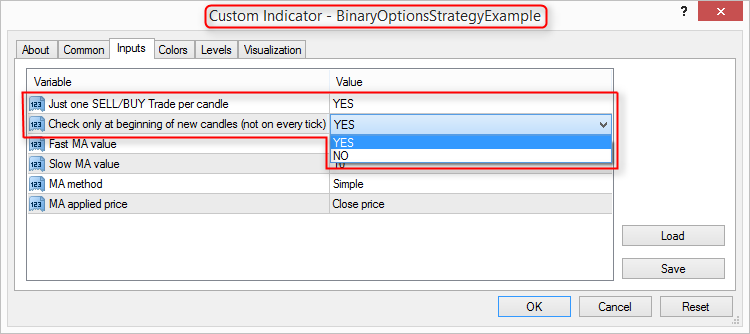### 3.2.3加上 CallStrategy()

...

//+------------------------------------------------------------------+
//| 自定义指标迭代函数                        |
//+------------------------------------------------------------------+
int OnCalculate(const int rates_total,
const int prev_calculated,
const datetime &time[],
const double &open[],
const double &high[],
const double &low[],
const double &close[],
const long &tick_volume[],
const long &volume[],
{
//---

CallStrategy(); //调用策略，函数位于 BinaryOptionsStrategyLibrary.mqh (上面已经包含了)

//--- 返回 prev_calculated 的值用于下一次调用

return
(rates_total);
}
//+------------------------------------------------------------------+

CallStrategy()- Binary-Options-Strategy-Library 中的这个函数将会调用在您指标中一个名为 CheckMyRules() 的函数，您可以在您的二元期权策略中设置您的条件，所以您必须在您的二元期权策略指标中实现 CheckMyRules() 函数。

### 3.2.4实现 CheckMyRules() 和辅助函数

...

//--- 输入参数
input int                period_fast        =  5;           //快速 MA 数值
input int                period_slow        = 10;           //慢速 MA 数值
input ENUM_MA_METHOD     method_both        = MODE_SMA;     //MA 方法
input ENUM_APPLIED_PRICE applied_price_both = PRICE_CLOSE;  //MA 应用的价格类型

...

//+------------------------------------------------------------------+
//| 在此设置您的交易规则 - 参见下面的例子.               |
//| StrategyTester 会调用这个函数来进行交易.           |
//| 不要:                               |
//| - 重命名函数                            |
//| - 增加函数的参数，比如 CheckMyRules(int a)            |
//| - 修改函数的返回值类型, 例如 int CheckMyRules()          |
//+------------------------------------------------------------------+
void CheckMyRules()
{

//保存 shift=0 (当前烛形) 的 MA 值 -> 当前烛形,
//调用辅助函数 GetValueForMA() 以取得数值 - 参见下面的辅助函数
double emaSlow_Current = GetValueForMA(period_slow, 0);
double emaFast_Current = GetValueForMA(period_fast, 0);

//保存 shift=1 (前一烛形) 的 MA 值-> 前一烛形,
//调用辅助函数 GetValueForMA() 以取得数值 - 参见下面的辅助函数
double emaSlow_Past = GetValueForMA(period_slow, 1);
double emaFast_Past = GetValueForMA(period_fast, 1);

if(emaFast_Past > emaSlow_Past
&& emaFast_Current < emaSlow_Past) //价差是否慢速MA和快速MA有交叉
{
}

if(emaFast_Past < emaSlow_Past
&& emaFast_Current > emaSlow_Past) //检查是否慢速 MA 和快速 MA 有交叉
{
}

}

//+------------------------------------------------------------------+
//| 在此设置您的辅助函数，参加下面的例子               |
//+------------------------------------------------------------------+
//----
//+------------------------------------------------------------------+
//| 根据周期数，方法，应用的价格和偏移取得 MA 数值。        |
//| iMA() 的详细信息参见 https://docs.mql4.com/indicators/ima    |
//+------------------------------------------------------------------+
double GetValueForMA(int _period,int _shift)
{
return iMA(NULL,0,_period,0,method_both,applied_price_both,_shift);
}

### 3.2.5打印出调试的数值

PrintDebugValue() 函数提供功能在测试器运行时打印出调试信息，在以下的例子中，MA 的数值和它们变量名称的标签会打印出来:

...

//--- 输入参数
input int                period_fast        =  5;           //快速 MA 数值
input int                period_slow        = 10;           //慢速 MA 数值
input ENUM_MA_METHOD     method_both        = MODE_SMA;     //MA 方法
input ENUM_APPLIED_PRICE applied_price_both = PRICE_CLOSE;  //MA 应用的价格类型

...

//+------------------------------------------------------------------+
//| 在此设置您的交易规则 - 参见下面的例子.               |
//| StrategyTester 会调用这个函数来进行交易.           |
//| 不要:                               |
//| - 重命名函数                            |
//| - 增加函数的参数，比如 CheckMyRules(int a)            |
//| - 修改函数的返回值类型, 例如 int CheckMyRules()          |
//+------------------------------------------------------------------+
void CheckMyRules()
{

//保存 shift=0 (当前烛形) 的 MA 值 -> 当前烛形,
//调用辅助函数 GetValueForMA() 以获取数值 - 参见下面的辅助函数
double emaSlow_Current = GetValueForMA(period_slow, 0);
double emaFast_Current = GetValueForMA(period_fast, 0);

//保存 shift=1 (前一烛形) 的 MA 值 -> 前一烛形,
//调用辅助函数 GetValueForMA() 以取得数值 - 参见下面的辅助函数
double emaSlow_Past = GetValueForMA(period_slow, 1);
double emaFast_Past = GetValueForMA(period_fast, 1);

PrintDebugValue("emaSlow_Current: ",(string)emaSlow_Current,0); //0行中的标签和数值
PrintDebugValue("emaFast_Current: ",(string)emaFast_Current,1); //1行中的标签和数值
PrintDebugValue("emaSlow_Past: ",(string)emaSlow_Past,2);       //2行中的标签和数值
PrintDebugValue("emaFast_Past: ",(string)emaFast_Past,3);       //3行中的标签和数值

if(emaFast_Past > emaSlow_Past
&& emaFast_Current < emaSlow_Past) //检查慢速 MA 和快速 MA 是否有交叉
{
}

if(emaFast_Past < emaSlow_Past
&& emaFast_Current > emaSlow_Past) //检查慢速 MA 和快速 MA 是否有交叉
{
}

}

//+------------------------------------------------------------------+
//| 在此设置您的辅助函数，参见下面的例子               |
//+------------------------------------------------------------------+
//----

//+------------------------------------------------------------------+
//| 根据周期数，方法，应用价格和偏移取得 MA 的值。       |
//| 关于 iMA()的详细信息参见 https://docs.mql4.com/indicators/ima  |
//+------------------------------------------------------------------+
double GetValueForMA(int _period,int _shift)
{
return iMA(NULL,0,_period,0,method_both,applied_price_both,_shift);
}

### 3.2.6使用外部指标(ex4 文件)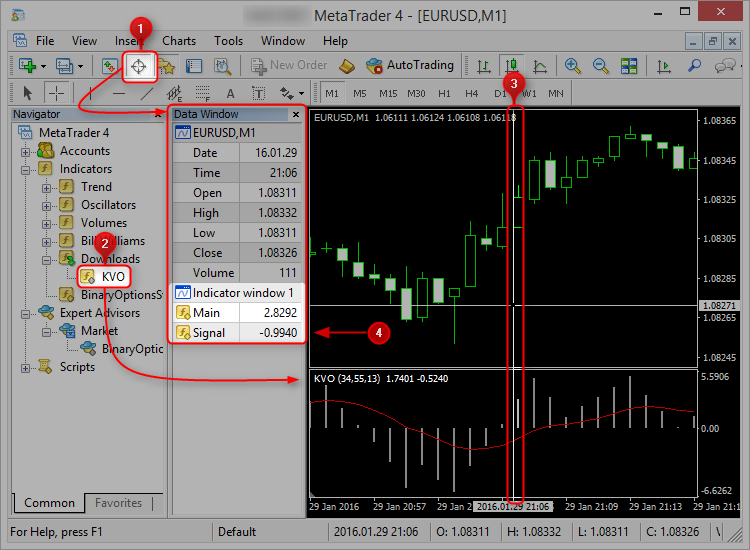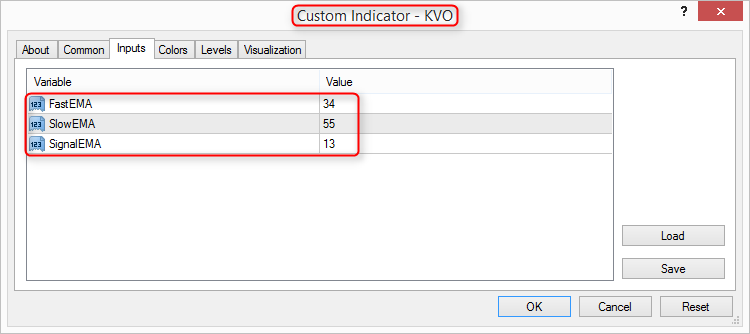...

//--- 输入参数
input int                period_fast        =  5;           //快速 MA 数值
input int                period_slow        = 10;           //慢速 MA 数值
input ENUM_MA_METHOD     method_both        = MODE_SMA;     //MA 方法
input ENUM_APPLIED_PRICE applied_price_both = PRICE_CLOSE;  //MA 应用的价格类型

...

//+------------------------------------------------------------------+
//| 在此设置您的交易规则 - 参见下面的例子.               |
//| StrategyTester 会调用这个函数来进行交易.           |
//| 不要:                               |
//| - 重命名函数                            |
//| - 增加函数的参数，比如 CheckMyRules(int a)            |
//| - 修改函数的返回值类型, 例如 int CheckMyRules()          |
//+------------------------------------------------------------------+
void CheckMyRules()
{

//保存 shift=0 (当前烛形) 的 MA 值 -> 当前烛形,
//调用辅助函数 GetValueForMA() 以获取数值 - 参见下面的辅助函数
double emaSlow_Current = GetValueForMA(period_slow, 0);
double emaFast_Current = GetValueForMA(period_fast, 0);

//保存 shift=1 (前一烛形) 的 MA 值 -> 前一烛形,
//调用辅助函数 GetValueForMA() 以取得数值 - 参见下面的辅助函数
double emaSlow_Past = GetValueForMA(period_slow, 1);
double emaFast_Past = GetValueForMA(period_fast, 1);

//保存 KVO 指标的当前烛形(偏移0)的信号值 (缓冲区 1)
double kvoSignal = GetValuesFromIndicator__KVO__(1,0);

PrintDebugValue("emaSlow_Current: ",(string)emaSlow_Current,0); //行0的标签和数值
PrintDebugValue("emaFast_Current: ",(string)emaFast_Current,1); //行1的标签和数值
PrintDebugValue("emaSlow_Past: ",(string)emaSlow_Past,2);       //行2的标签和数值
PrintDebugValue("emaFast_Past: ",(string)emaFast_Past,3);       //行3的标签和数值

if(emaFast_Past > emaSlow_Past
&& emaFast_Current < emaSlow_Past //检查是否慢速 MA 和快速 MA 有交叉
&& kvoSignal < 0) //检查 KVO 信号值是否小于 0
{
}

if(emaFast_Past < emaSlow_Past
&& emaFast_Current > emaSlow_Past //检查慢速 MA 和快速 MA 是否有交叉
&& kvoSignal > 0) //检查 KVO 信号值是否大于 0
{
}

}

//+------------------------------------------------------------------+
//| 在此设置您的辅助函数，参见下面的例子               |
//+------------------------------------------------------------------+
//----

//+------------------------------------------------------------------+
//| 根据周期数，方法，应用价格和偏移取得 MA 的数值.        |
//| iMA() 的详细信息参见 https://docs.mql4.com/indicators/ima   |
//+------------------------------------------------------------------+
double GetValueForMA(int _period,int _shift)
{
return iMA(NULL,0,_period,0,method_both,applied_price_both,_shift);
}

//+------------------------------------------------------------------+
//| 如何从外部指标中取得数值的例子               |
//| 参见 https://docs.mql4.com/indicators/icustom          |
//| 参数:                                                      |
//| int _buffer - 指标缓冲区 (从 0 开始)                |
//| int _shift - 偏移值; 0 = 当前柱, 1 = 前一柱 |
//+------------------------------------------------------------------+
double GetValuesFromIndicator__KVO__(int _buffer, int _shift=0) //把 "__KVO__" 改成指标名称
{

return (
iCustom (
NULL,                      //NULL 表示测试器选择的当前时段 - 不需改变
0,                         //0 表示测试器中选择的当前交易品种 - 不需要改变

//开始编辑
//开始指标的输入参数
34,
55,
13,
//指标输入参数结束
//结束编辑

_buffer,                   //缓冲区索引 (从0开始), _buffer 是通过函数参数传入的 - 不需要修改
_shift                     //偏移 (0 表示当前烛形), _shift 是通过函数参数传入的 - 不需要改变
)
);

}

### 3.3完整代码

//+------------------------------------------------------------------+
//|                                 BinaryOptionsStrategyExample.mq4 |
//|                         https://www.mql5.com/en/users/__martin__ |
//+------------------------------------------------------------------+
#property version   "1.00"
#property strict
#property indicator_separate_window

#include <BinaryOptionsStrategyLibrary.mqh>

//+------------------------------------------------------------------+
//| 把您的输入参数放到这里 - 参见下面的例子             |
//+------------------------------------------------------------------+
//--- 输入参数
input int                period_fast        =  5;           //快速 MA 数值
input int                period_slow        = 10;           //慢速 MA 数值
input ENUM_MA_METHOD     method_both        = MODE_SMA;     //MA 方法
input ENUM_APPLIED_PRICE applied_price_both = PRICE_CLOSE;  //MA 应用的价格

//+------------------------------------------------------------------+
//| 自定义指标初始化函数                        |
//+------------------------------------------------------------------+
int OnInit()
{
//--- 指标缓冲区的映射

//---
return(INIT_SUCCEEDED);
}

//+------------------------------------------------------------------+
//| 自定义指标迭代函数                        |
//+------------------------------------------------------------------+
int OnCalculate(const int rates_total,
const int prev_calculated,
const datetime &time[],
const double &open[],
const double &high[],
const double &low[],
const double &close[],
const long &tick_volume[],
const long &volume[],
{
//---

CallStrategy(); //调用策略，函数位于 BinaryOptionsStrategyLibrary.mqh (上面已经包含)

//--- 返回 prev_calculated 的值用于下次调用
return(rates_total);
}

//+------------------------------------------------------------------+
//| 把您的交易规则放到这里 - 参见下面的例子.               |
//| StrategyTester 会调用这个函数来设置交易.           |
//| 不要:                                                          |
//| - 重新命名函数                            |
//| - 增加函数参数，例如 CheckMyRules(int a)              |
//| - 修改函数返回值类型, 例如 int CheckMyRules()           |
//+------------------------------------------------------------------+
void CheckMyRules()
{

//保存 shift=0 (当前烛形) 的 MA 值 -> 当前烛形,
//调用辅助函数 GetValueForMA() 来取得数值 - 参见下面的辅助函数
double emaSlow_Current = GetValueForMA(period_slow, 0);
double emaFast_Current = GetValueForMA(period_fast, 0);

//保存 shift=1 (前一烛形) 的 MA 值 -> 前一烛形,
//调用辅助函数 GetValueForMA() 来取得数值 - 参见下面的辅助函数
double emaSlow_Past = GetValueForMA(period_slow, 1);
double emaFast_Past = GetValueForMA(period_fast, 1);

//保存KVO指标的当前烛形(偏移0)的信号值 (缓冲区1)
double kvoSignal = GetValuesFromIndicator__KVO__(1,0);

PrintDebugValue("emaSlow_Current: ",(string)emaSlow_Current,0); //行0的标签和数值
PrintDebugValue("emaFast_Current: ",(string)emaFast_Current,1); //行1的标签和数值
PrintDebugValue("emaSlow_Past: ",(string)emaSlow_Past,2);       //行2的标签和数值
PrintDebugValue("emaFast_Past: ",(string)emaFast_Past,3);       //行3的标签和数值

if(emaFast_Past > emaSlow_Past
&& emaFast_Current < emaSlow_Past    //检查慢速 MA 和快速 MA 是否有交叉
&& kvoSignal < 0)                    //检查 KVO 信号值是否小于 0
{
}

if(emaFast_Past < emaSlow_Past
&& emaFast_Current > emaSlow_Past    //检查是否慢速 MA 和快速 MA 有交叉
&& kvoSignal > 0)                    //检查 KVO 信号值是否大于 0
{
}

}

//+------------------------------------------------------------------+
//| 在此设置您的辅助函数，参见下面的例子               |
//+------------------------------------------------------------------+
//----

//+------------------------------------------------------------------+
//| 根据周期数，方法，应用价格和偏移取得 MA 的值，        |
//| 关于 iMA() 的详细信息参见 https://docs.mql4.com/indicators/ima  |
//+------------------------------------------------------------------+
double GetValueForMA(int _period,int _shift)
{
return iMA(NULL,0,_period,0,method_both,applied_price_both,_shift);
}

//+------------------------------------------------------------------+
//| 如何从外部指标中取得数值的例子,               |
//| 参见 https://docs.mql4.com/indicators/icustom          |
//| 参数:                               |
//| int _buffer - 指标缓冲区 (从 0 开始)               |
//| int _shift - 偏移值; 0 = 当前烛形, 1 = 前一烛形 |
//+------------------------------------------------------------------+
double GetValuesFromIndicator__KVO__(int _buffer, int _shift=0) //把 "__KVO__" 改成指标名称
{
return (
iCustom (
NULL,                      //NULL 表示测试器中选择的当前时段 - 不需要改变
0,                         //0 表示测试器中选择的当前交易品种 - 不需要改变

//开始编辑
//开始指标输入参数
34,
55,
13,
//指标输入参数结束
//结束编辑

_buffer,                   //缓冲区索引 (从0开始), _buffer 是通过函数参数传入的 - 不需要改变
_shift                     //偏移 (0 表示当前烛形), _shift 是通过函数参数传入的 - 不需要改变
)
);
}
//+-----------------------------------------------------------------+

### 4. 运行一次回溯测试 (视频)

• 在 MetaTrader 4 中的策略测试器中启动 Binary-Options-Strategy-Tester 并设置输入参数
• 把您的二元期权策略指标拖到图表上，设置输入参数并在"通用"页面选择 "允许外部EA交易输入"
• 把您使用的指标使用它们的输入参数拖到图表上，在测试器运行的时候观察它们的数值(可选)
• 在模板中保存所有的设置并使用所有的设置再次运行测试 - 使用策略测试器的暂停按钮(可选)
• 在策略测试器图表上查看您二元期权策略的结果

### 5. 运行一次前瞻测试

• 把 Binary-Options-Strategy-Tester 工具拖到模拟或者真实帐户图表中并设置输入参数
• 把您的二元期权策略指标拖到图表上，设置输入参数并在"通用"页面选择 "允许外部EA交易输入"
• 把您使用的指标使用它们的输入参数拖到图表上，当前瞻测试正在运行时观察它们的数值（可选）
• 把全部设置保存到模板中并再次使用全部设置运行测试 (可选)
• 在模拟或者真实帐户图表中查看您二元期权策略的结果

### 7. 杂项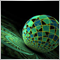采用栈式 RBM 的深度神经网络。自训练, 自控制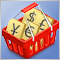在外汇市场中货币篮子的运作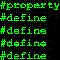在非标准时间范围上测试 Expert Advisor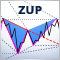ZUP - 通用之字折线构造 Pesavento 形态。图形界面